# CIE A Level Chemistry复习笔记1.2.7 Reacting Masses & Volumes

### Mole Calculations

• The number of moles of a substance can be found by using the following equation:• It is important to be clear about the type of particle you are referring to when dealing with moles
• Eg. 1 mole of CaF2 contains one mole of CaFformula units, but one mole of Ca2+ and two moles of F- ions

#### Reacting masses

• The masses of reactants are useful to determine how much of the reactants exactly react with each other to prevent waste
• To calculate the reacting masses, the chemical equation is required
• This equation shows the ratio of moles of all the reactants and products, also called the stoichiometry, of the equation
• To find the mass of products formed in a reaction the following pieces of information is needed:
• The mass of the reactants
• The molar mass of the reactants
• The balanced equation

#### Percentage yield

• In a lot of reactions, not all reactants react to form products which can be due to several factors:
• Other reactions take place simultaneously
• The reaction does not go to completion
• Reactants or products are lost to the atmosphere
• The percentage yield shows how much of a particular product you get from the reactants compared to the maximum theoretical amount that you can get:• Where actual yield is the number of moles or mass of product obtained experimentally
• The predicted yield is the number of moles or mass obtained by calculation

#### Worked example: Mass calculation using moles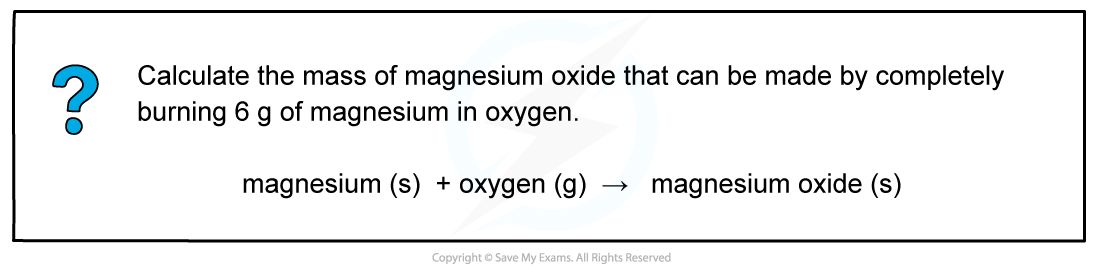• Step 1: The symbol equation is:

2Mg(s)    +     O2(g)      →      2MgO(s)

• Step 2: The relative formula masses are:

Magnesium : 24         Oxygen : 32         Magnesium Oxide : 40

• Step 3: Calculate the moles of magnesium used in reaction• Step 4: Find the ratio of magnesium to magnesium oxide using the balanced chemical equationTherefore, 0.25 mol of MgO is formed

• Step 5: Find the mass of magnesium oxide

mass = mol x Mr

mass = 0.25 mol x 40 g mol-1mass = 10 g

Therefore, mass of magnesium oxide produced is 10 g

#### Worked Example: Calculate % yield using moles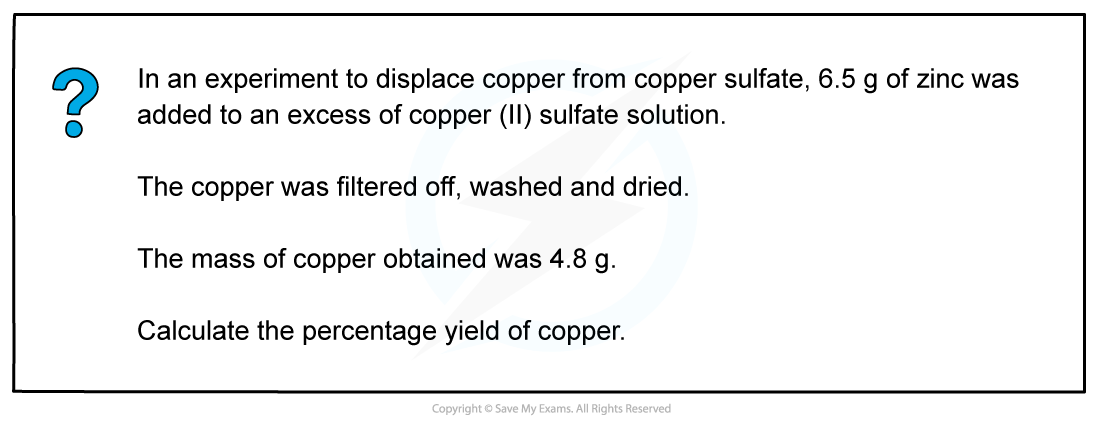• Step 1: The symbol equation is:

Zn (s)    +    CuSO4 (aq)     →     ZnSO4 (aq)    + Cu (s)

•  Step 2: Calculate the amount of zinc reacted in moles• Step 3: Calculate the maximum amount of copper that could be formed from the molar ratio:

Since the ratio of Zn(s) to Cu(s) is 1:1 a maximum of 0.10 moles can be produced

• Step 4: Calculate the maximum mass of copper that could be formed (theoretical yield)

mass =   mol   x   Mr

mass =   0.10 mol x 64 g mol-1

mass =   6.4 g

• Step 5: Calculate the percentage yield of copper#### Excess & limiting reagents

• Sometimes, there is an excess of one or more of the reactants (excess reagent)
• The reactant which is not in excess is called the limiting reagent
• To determine which reactant is limiting:
• The number of moles of the reactants should be calculated
• The ratio of the reactants shown in the equation should be taken into account eg:

C  + 2H2      →     CH4

There are 10 mol of Carbon reacting with 3 mol of Hydrogen

• Hydrogen is the limiting reagent and since the ratio of C : H2 is 1:2 only 1.5 mol of C will react with 3 mol of H2

#### Worked example: Excess & limiting reagent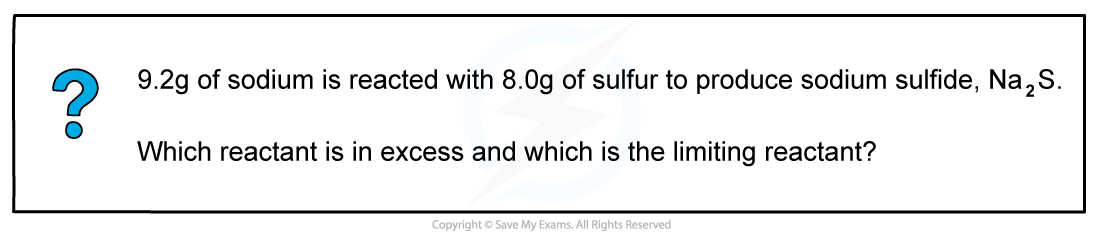• Step 1: Calculate the moles of each reactant• Step 2: Write the balanced equation and determine the molar ratio

2Na + S → Na2S

The molar ratio of Na: Na2S is 2:1

• Step 3: Compare the moles and determine the limiting reagent

So to react completely 0.40 moles of Na require 0.20 moles of S and since there are 0.25 moles of S, then S is in excess. Na is therefore the limiting reactant.

#### Volumes of gases

• Avogadro suggested that ‘equal volumes of gases contain the same number of molecules’ (also called Avogadro’s hypothesis)
• At room temperature (20 degrees Celsius) and pressure (1 atm) one mole of any gas has a volume of 24.0 dm3
• This molar gas volume of 24.0 dm3 can be used to find:
• The volume of a given mass or number of moles of gas:

volume of gas (dm3) = amount of gas (mol) x 24

• The mass or number of moles of a given volume of gas:#### Worked example: Calculation volume of gas using excess & limiting reagents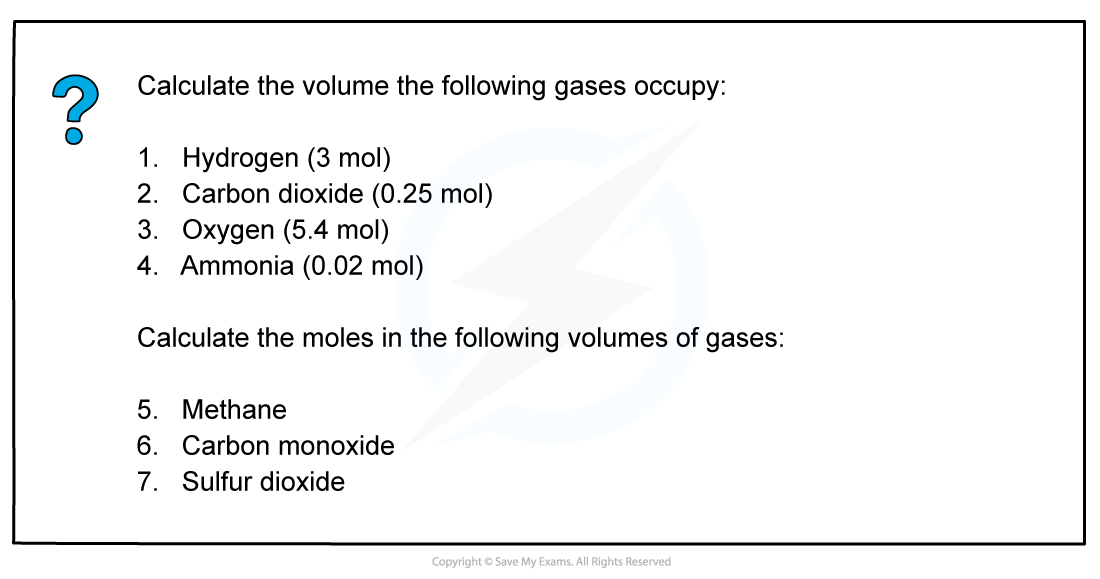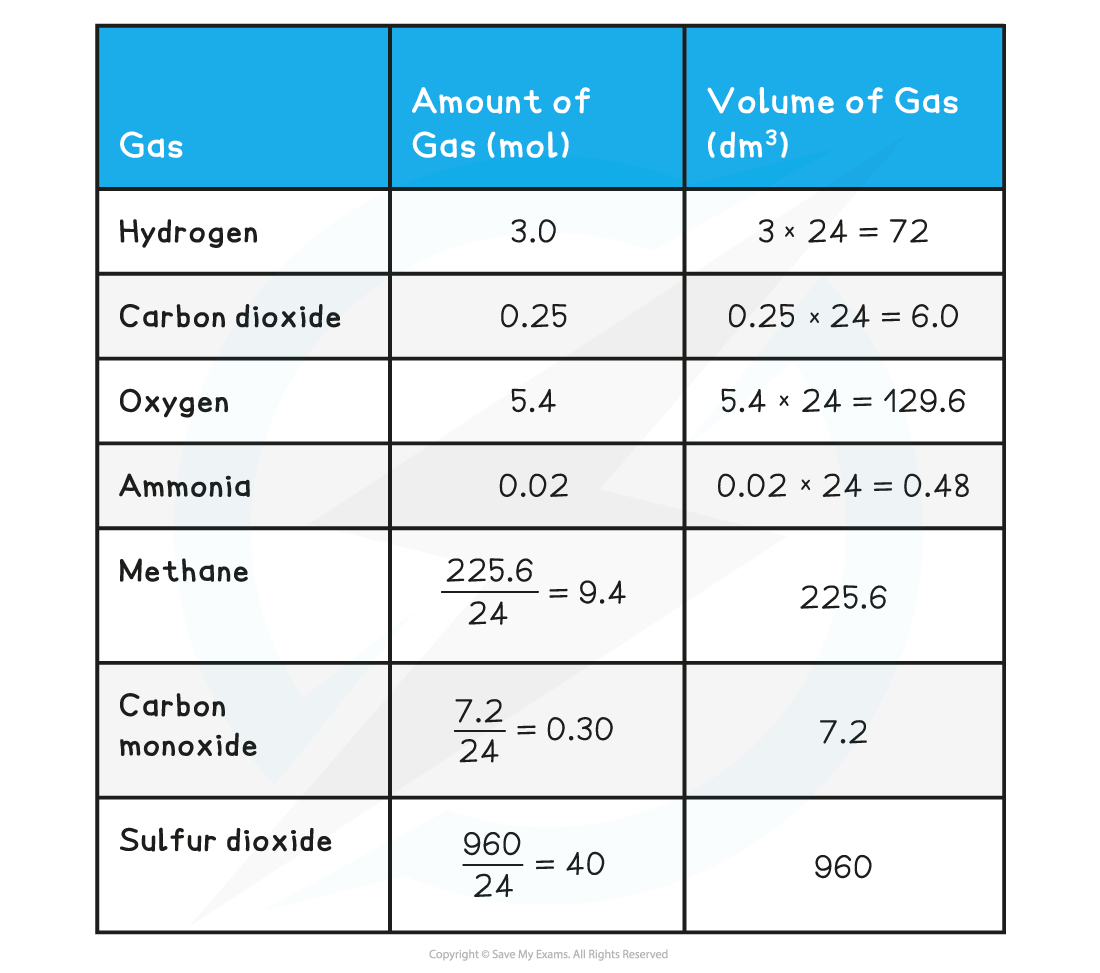#### Volumes & concentrations of solutions

• The concentration of a solution is the amount of solute dissolved in a solvent to make 1 dm3 of  solution
• The solute is the substance that dissolves in a solvent to form a solution
• The solvent is often water• A concentrated solution is a solution that has a high concentration of solute
• A dilute solution is a solution with a low concentration of solute
• When carrying out calculations involve concentrations in mol dm-3 the following points need to be considered:
• Change mass in grams to moles
• Change cm3 to dm3
• To calculate the mass of a substance present in solution of known concentration and volume:
• Rearrange the concentration equation

number of moles (mol) = concentration (mol dm-3) x volume (dm3)

• Multiply the moles of solute by its molar mass

mass of solute (g) = number of moles (mol) x molar mass (g mol-1)

#### Worked example: Calculating volume from concentration• Step 1: Write the balanced symbol equation

CaCO3  +  2HCl  →  CaCl2  +  H2O  +  CO2

• Step 2: Calculate the amount, in moles, of calcium carbonate that reacts• Step 3: Calculate the moles of hydrochloric acid required using the reaction’s stoichiometry

1 mol of CaCO3 requires 2 mol of HCl

So 0.025 mol of CaCO3 requires 0.05 mol of HCl

• Step 4: Calculate the volume of HCl requiredVolume of hydrochloric acid = 0.05 dm3

#### Worked example: Neutralisation calculation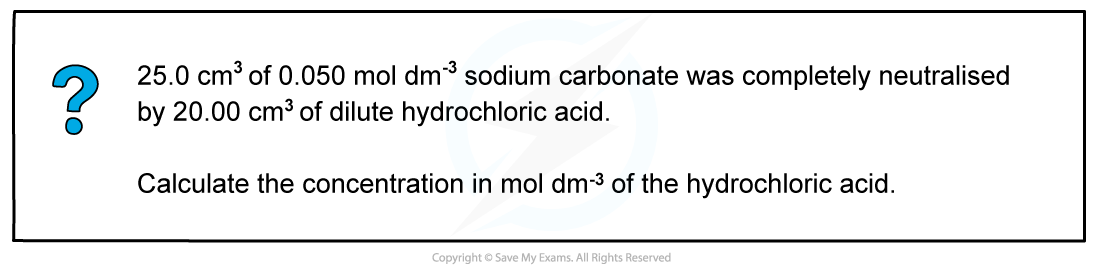• Step 1: Write the balanced symbol equation

Na2CO3  +  2HCl  →  Na2Cl2  +  H2O  +  CO2

• Step 2: Calculate the amount, in moles, of sodium carbonate reacted by rearranging the equation for amount of substance (mol) and dividing by the volume by 1000 to convert cm3 to dm3

amount (Na2CO3) = 0.025 dm3 x 0.050 mol dm-3 = 0.00125 mol

• Step 3: Calculate the moles of hydrochloric acid required using the reaction’s stoichiometry

1 mol of Na2CO3 reacts with 2 mol of HCl, so the molar ratio is 1 : 2

Therefore 0.00125 moles of Na2CO3 react with 0.00250 moles of HCl

• Step 4: Calculate the concentration, in mol dm-3 of hydrochloric acidconcentration (HCl) (mol dm-3) = 0.125 mol dm-3

#### Stoichiometric relationships

• The stoichiometry of a reaction can be found if the exact amounts of reactants and products formed are known
• The amounts can be found by using the following equation:• The gas volumes can be used to deduce the stoichiometry of a reaction
• Eg. in the combustion of 50 cm3 of propane reacting with 250 cm3 of oxygen, 150 cm3  of carbon dioxide is formed suggesting that the ratio of propane:oxygen:carbon dioxide is 1:5:3

C3H8 (g) + 5O2 (g) → 3CO2 (g) + H2O (l)